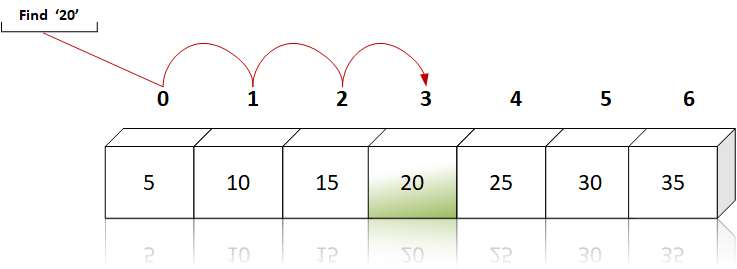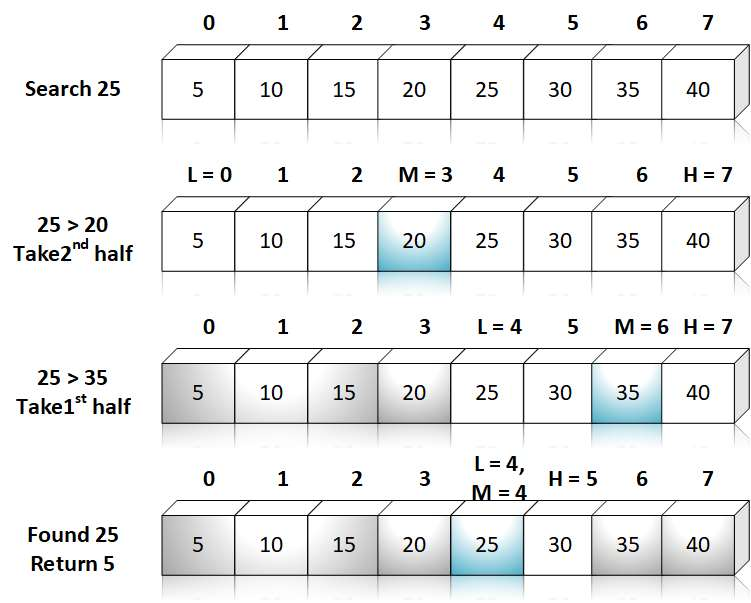# Array C Programing

Answer: Searching is the process of finding the location of given element in the linear array. The search is said to be successful if the given element is found i.e. the element does exists in the array; otherwise unsuccessful. There arce two techniques for searching.

• Linear Search or Sequential Search
• Binary Search

If the elements are in random order, then one have to use linear search technique. Given no information about the array a, the only way to search for given element item is to compare item with each element of a one by one.

This method, which traverses a sequentially to locate item is called linear search or sequential search. The following code shows the implementation of linear search.``````
int LinearSearch(int a, int n, int item)
{
int k;
for (k = 0; k < n; k++)
{
if (a[k] == item)
return k;
}
}

``````

Binary search is used when the array is sorted. In this we divide the array into half and compare it with the element item if the mid value is less than item the value is in second half, otherwise, it is in first half.

The following code shows the implementation of binary search``````
int LinearSearch(int a, int n, int item)
{
int beg, end, mid;
beg = 0; end = n - 1;
mid = (beg + end) / 2;
while ((beg <= end) && (a[mid] != item))
{
if (item < a[mid])
end = mid - 1;
else
beg = mid + 1;
mid = (beg + end) / 2;
}
}

``````